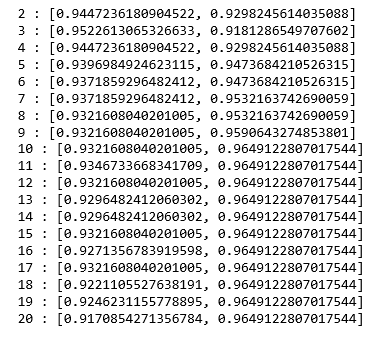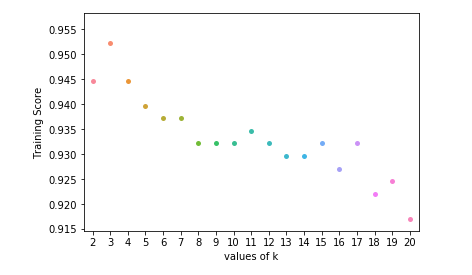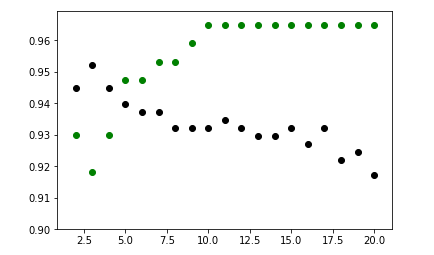# ML | Implementation of KNN classifier using Sklearn

Prerequisite: K-Nearest Neighbours Algorithm

K-Nearest Neighbors is one of the most basic yet essential classification algorithms in Machine Learning. It belongs to the supervised learning domain and finds intense application in pattern recognition, data mining and intrusion detection. It is widely disposable in real-life scenarios since it is non-parametric, meaning, it does not make any underlying assumptions about the distribution of data (as opposed to other algorithms such as GMM, which assume a Gaussian distribution of the given data).

This article will demonstrate how to implement the K-Nearest neighbors classifier algorithm using Sklearn library of Python.

Step 1: Importing the required Libraries

 `import` `numpy as np ` `import` `pandas as pd ` `from` `sklearn.model_selection ``import` `train_test_split ` `from` `sklearn.neighbors ``import` `KNeighborsClassifier ` `import` `matplotlib.pyplot as plt  ` `import` `seaborn as sns `

 `cd C:\Users\Dev\Desktop\Kaggle\Breast_Cancer ` `# Changing the read file location to the location of the file ` ` `  `df ``=` `pd.read_csv(``'data.csv'``) ` ` `  `y ``=` `df[``'diagnosis'``] ` `X ``=` `df.drop(``'diagnosis'``, axis ``=` `1``) ` `X ``=` `X.drop(``'Unnamed: 32'``, axis ``=` `1``) ` `X ``=` `X.drop(``'id'``, axis ``=` `1``) ` `# Separating the dependent and independent variable ` ` `  `X_train, X_test, y_train, y_test ``=` `train_test_split( ` `             ``X, y, test_size ``=` `0.3``, random_state ``=` `0``) ` `# Splitting the data into training and testing data `

Step 3: Training the model

 `K ``=` `[] ` `training ``=` `[] ` `test ``=` `[] ` `scores ``=` `{} ` ` `  `for` `k ``in` `range``(``2``, ``21``): ` `    ``clf ``=` `KNeighborsClassifier(n_neighbors ``=` `k) ` `    ``clf.fit(X_train, y_train) ` ` `  `    ``training_score ``=` `clf.score(X_train, y_train) ` `    ``test_score ``=` `clf.score(X_test, y_test) ` `    ``K.append(k) ` ` `  `    ``training.append(training_score) ` `    ``test.append(test_score) ` `    ``scores[k] ``=` `[training_score, test_score] `

Step 4: Evaluating the model

 `for` `keys, values ``in` `scores.items(): ` `    ``print``(keys, ``':'``, values) `We now try to find the optimum value for ‘k’ ie the number of nearest neighbors.

Step 5: Plotting the training and test scores graph

 `ax ``=` `sns.stripplot(K, training); ` `ax.``set``(xlabel ``=``'values of k'``, ylabel ``=``'Training Score'``)   ` ` `  `plt.show() ` `# function to show plot ``ax ``=` `sns.stripplot(K, test); ` `ax.``set``(xlabel ``=``'values of k'``, ylabel ``=``'Test Score'``) ` `plt.show() ``plt.scatter(K, training, color ``=``'k'``) ` `plt.scatter(K, test, color ``=``'g'``) ` `plt.show() ` `# For overlapping scatter plots `From the above scatter plot, we can come to the conclusion that the optimum value of k will be around 5.

Whether you're preparing for your first job interview or aiming to upskill in this ever-evolving tech landscape, GeeksforGeeks Courses are your key to success. We provide top-quality content at affordable prices, all geared towards accelerating your growth in a time-bound manner. Join the millions we've already empowered, and we're here to do the same for you. Don't miss out - check it out now!

Previous
Next